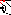# MINLPLib

### A Library of Mixed-Integer and Continuous Nonlinear Programming Instances

#### Instance synthes2

 Formatsⓘ ams gms mod nl osil py Primal Bounds (infeas ≤ 1e-08)ⓘ 73.03531253 p1 ( gdx sol ) (infeas: 1e-13) Other points (infeas > 1e-08)ⓘ Dual Boundsⓘ 73.03504110 (ALPHAECP)73.03531245 (ANTIGONE)73.03531245 (BARON)73.03531252 (BONMIN)73.03531253 (COUENNE)73.03531253 (LINDO)73.03530997 (SCIP)73.03529225 (SHOT) Referencesⓘ Duran, Marco A and Grossmann, I E, An Outer-Approximation Algorithm for a Class of Mixed-integer Nonlinear Programs, Mathematical Programming, 36:3, 1986, 307-339. Sourceⓘ MacMINLP model synthes2.mod Applicationⓘ Synthesis of processing system Added to libraryⓘ 01 May 2001 Problem typeⓘ MBNLP #Variablesⓘ 11 #Binary Variablesⓘ 5 #Integer Variablesⓘ 0 #Nonlinear Variablesⓘ 4 #Nonlinear Binary Variablesⓘ 0 #Nonlinear Integer Variablesⓘ 0 Objective Senseⓘ min Objective typeⓘ nonlinear Objective curvatureⓘ convex #Nonzeros in Objectiveⓘ 11 #Nonlinear Nonzeros in Objectiveⓘ 4 #Constraintsⓘ 14 #Linear Constraintsⓘ 11 #Quadratic Constraintsⓘ 0 #Polynomial Constraintsⓘ 0 #Signomial Constraintsⓘ 0 #General Nonlinear Constraintsⓘ 3 Operands in Gen. Nonlin. Functionsⓘ exp log Constraints curvatureⓘ convex #Nonzeros in Jacobianⓘ 37 #Nonlinear Nonzeros in Jacobianⓘ 4 #Nonzeros in (Upper-Left) Hessian of Lagrangianⓘ 6 #Nonzeros in Diagonal of Hessian of Lagrangianⓘ 4 #Blocks in Hessian of Lagrangianⓘ 3 Minimal blocksize in Hessian of Lagrangianⓘ 1 Maximal blocksize in Hessian of Lagrangianⓘ 2 Average blocksize in Hessian of Lagrangianⓘ 1.333333 #Semicontinuitiesⓘ 0 #Nonlinear Semicontinuitiesⓘ 0 #SOS type 1ⓘ 0 #SOS type 2ⓘ 0 Minimal coefficientⓘ 2.0000e-01 Maximal coefficientⓘ 6.0000e+01 Infeasibility of initial pointⓘ 1 Sparsity JacobianⓘSparsity Hessian of Lagrangianⓘ```\$offlisting
*
*  Equation counts
*      Total        E        G        L        N        X        C        B
*         15        2        0       13        0        0        0        0
*
*  Variable counts
*                   x        b        i      s1s      s2s       sc       si
*      Total     cont   binary  integer     sos1     sos2    scont     sint
*         12        7        5        0        0        0        0        0
*  FX      0
*
*  Nonzero counts
*      Total    const       NL      DLL
*         49       41        8        0
*
*  Solve m using MINLP minimizing objvar;

Variables  x1,x2,x3,x4,x5,x6,b7,b8,b9,b10,b11,objvar;

Positive Variables  x1,x2,x3,x4,x5,x6;

Binary Variables  b7,b8,b9,b10,b11;

Equations  e1,e2,e3,e4,e5,e6,e7,e8,e9,e10,e11,e12,e13,e14,e15;

e1.. -log(1 + x4 + x5) =L= 0;

e2.. exp(x1) - 10*b7 =L= 1;

e3.. exp(0.833333*x2) - 10*b8 =L= 1;

e4..    1.25*x3 - 10*b9 =L= 0;

e5..    x4 + x5 - 10*b10 =L= 0;

e6..  - 2*x3 + 2*x6 - 10*b11 =L= 0;

e7..  - x1 - x2 - 2*x3 + x4 + 2*x6 =L= 0;

e8..  - x1 - x2 - 0.75*x3 + x4 + 2*x6 =L= 0;

e9..    x3 - x6 =L= 0;

e10..    2*x3 - x4 - 2*x6 =L= 0;

e11..  - 0.5*x4 + x5 =L= 0;

e12..  - 0.2*x4 - x5 =L= 0;

e13..    b7 + b8 =E= 1;

e14..    b10 + b11 =L= 1;

e15.. -(exp(x1) - 10*x1 + exp(0.833333*x2) - 15*x2 - 60*log(1 + x4 + x5) + 15*
x4 + 5*x5) + 15*x3 + 20*x6 - 5*b7 - 8*b8 - 6*b9 - 10*b10 - 6*b11 + objvar
=E= 140;

* set non-default bounds
x1.up = 2;
x2.up = 2;
x3.up = 2;
x6.up = 3;

Model m / all /;

m.limrow=0; m.limcol=0;
m.tolproj=0.0;

\$if NOT '%gams.u1%' == '' \$include '%gams.u1%'

\$if not set MINLP \$set MINLP MINLP
Solve m using %MINLP% minimizing objvar;

```

Last updated: 2023-06-01 Git hash: fdbb0df7